# Why is displacement current difficult to measure?

• Felipe Lincoln
Inside a wire we also have a displacement current due to its electric field given by ##E=V/x##, so in this specific case the magnetic field generated by this displacement current is much lower, since now we have ##i_d << i## right?Yes, in principle the magnetic field generated by displacement current in a capacitor is more difficult to measure.f

#### Felipe Lincoln

Gold Member
I had a question in my textbook asking why is the magnetic field generated by the displacement current in a capacitor more difficult to be measured than the magnetic field generated by the current in the wire. But I don't know how is this type of magnetic field measured, I only know that they have to be the same in either case because the current in the wire is the same as the displacement current, therefore by Ampere's law they have to be the same. (In a region out of the capacitor!)

Where would you put the measurement device in the two cases? How well does that work?

•sophiecentaur and Felipe Lincoln
I would guess this is to do with magnitude of the field. The relevant law here is:

##\nabla\times \vec{B}=\frac{\dot{\vec{E}}}{c^2}##

Where ##\vec{B}## is magnetic field, ##c## is the speed of light and ##\vec{E}## is electric field. Let electric field be uniform, but time-varying, within the considered region (deeply sub-wavelength regime) ##\vec{E}=\vec{\hat{z}} E_0 \cos\omega t##. It follows that the azimuthal magnetic field is on the scale of:

##B \sim \frac{\omega r E_0}{2 c^2}##

where ##\omega## is the angular frequency and ##r=\sqrt{x^2+y^2}## is how far your detector is from the z-axis. So taking reasonable values ##r=1\,\mbox{cm}##, ##E_0=1\,\mbox{kV/m}##, ##\omega=2\pi\times1\,{kHz}##

##B\approx3\,\mbox{pT}##

This is very small, and I was very generous with electric field strength and frequency. You can detect such fields, but only with very specialized detectors (SQUIDs and NV centres for example).

EDIT: come to think of it, ##E=1\,\mbox{kV/m}## is not too big in a small capacitor (1 V/mm), but then you have no place to put your detector, as noted by mfb

Last edited:
•mfb and Felipe Lincoln
Right, but I can't see the difference between the methods I'd have to use for both cases. As displacement current behaves like current (outside of the wire and capacitor) I don't see why I should use different approach on detecting them.

why is the magnetic field generated by the displacement current in a capacitor more difficult to be measured than the magnetic field generated by the current in the wire.
The Field due to the current in the wire would be a lot greater and, so, would make it difficult to measure, I think. That factor of 1/c2 is pretty relevant.

•Felipe Lincoln
I'll try to explain better my confusion:
## \oint_C\vec{B}\cdot\vec{dl}=\mu_0(i+i_d)## such that ##C## is a curve with radius great enough to be out of the wire and out of the capacitor.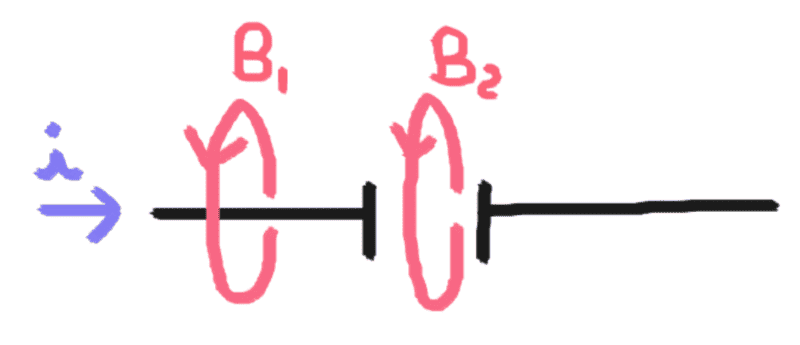We all agree that ##i=i_d## right ? By this reason, following the equation I wrote above, why would ##B_1 \neq B_2## and therefore, why would ##B_2## be more difficult to be measured?

#### Attachments

The Field due to the current in the wire would be a lot greater and, so, would make it difficult to measure, I think. That factor of 1/c2 is pretty relevant.
Inside a wire we also have a displacement current due to its electric field given by ##E=V/x##, so in this specific case the magnetic field generated by this displacement current is much lower, since now we have ##i_d << i## right?

why would ##B_1 \neq B_2## and therefore, why would ##B_2## be more difficult to be measured?

I suggest simply posing the problem and solving it mathematically. Take parallel-plate capacitor with very large plates which is fed by very thin wires. Take a contour around the current-carrying section. If the current is large controur is small you can ignore the electric field and use ##\nabla\times\vec{B}=\mu_0 \vec{J}##, and you will get one value for magnetic field. Next take a contour inside the capacitor, use ##\nabla\times\vec{B}=\dot{\vec{E}}/c^2##, get the second value. Then compare.

Right, but I can't see the difference between the methods I'd have to use for both cases. As displacement current behaves like current (outside of the wire and capacitor) I don't see why I should use different approach on detecting them.

"In theory there is no difference between theory and practice, but in practice there is" :-)

The types of detectors would have to be different, indeed there might not even be a detector that fits all the criteria. But, in principle, yes, displacement currents produce magnetic fields.

I'll try to explain better my confusion:
## \oint_C\vec{B}\cdot\vec{dl}=\mu_0(i+i_d)## such that ##C## is a curve with radius great enough to be out of the wire and out of the capacitor.
[

By the way your picture is somewhat messed up. Either you make your second contour much smaller than capacitor plates, then you have simple uniform electric field, i. e. displacement current, or you make your contour large, but the electric field now becomes all complicated (due to edges of the capacitor). In the first case you will measure small magnetic field because you only capture part of the 'displacement current', in the second case the magnetic field is small because you are far away from the wire. Take your pick.

Last edited:
second case the magnetic field is small because you are far away from the wire. Take your pick.
I agree, it is small, but is it different than the magnetic field measured at the same distance in the current part?

I suggest simply posing the problem and solving it mathematically.
:-)

Well, I did and it told me it is equal.
So returning to the main question. Why is it more difficult to be measured?

From the RF engineering perspective. I would say it's because most physical electronic capacitors do not to radiate. The closely-spaced plates means the electric filed coupling between the two halves approaches 100% so the displacement current term is very low.

The physical capacitors that do radiate because the capacitive element separation is a sizable fraction of the time varying signals wavelength are normally called antennas.

Of course, the displacement current is not a current at all. It is, in fact, associated with the generation of magnetic fields by time-varying electric fields. Maxwell came up with this rather curious name because many of his ideas regarding electric and magnetic fields were completely wrong. For instance, Maxwell believed in the æther, and he thought that electric and magnetic fields were some sort of stresses in this medium. He also thought that the displacement current was associated with displacements of the æther (hence, the name). The reason that these misconceptions did not invalidate his equations is quite simple. Maxwell based his equations on the results of experiments, and he added in his extra term so as to make these equations mathematically self-consistent. Both of these steps are valid irrespective of the existence or non-existence of the æther.
...
So,'' you might say, why did you bother mentioning this displacement current thing in the first place if it is undetectable?'' Again, a perfectly fair question. The answer is that the displacement current is detectable in some experiments. Suppose that we take an FM radio signal, amplify it so that its peak voltage is one hundred volts, and then apply it to the parallel plate capacitor in the previous hypothetical experiment. What size of magnetic field would this generate? Well, a typical FM signal oscillates at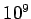Hz, so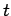in the previous example changes from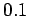seconds to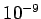seconds. Thus, the induced magnetic field is about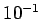gauss. This is certainly detectable by modern technology. So, it would seem that if the electric field is oscillating fast then electric induction of magnetic fields is an observable effect. In fact, there is a virtually infallible rule for deciding whether or not the displacement current can be neglected in Eq. (413). If electromagnetic radiation is important then the displacement current must be included. On the other hand, if electromagnetic radiation is unimportant then the displacement current can be safely neglected. Clearly, Maxwell's inclusion of the displacement current in Eq. (413) was a vital step in his later realization that his equations allowed propagating wave-like solutions. These solutions are, of course, electromagnetic waves. But, more of this later.

http://farside.ph.utexas.edu/teaching/em/lectures/node46.html

#### Attachments

•Cryo, vsv86 and Felipe Lincoln
Well, I did and it told me it is equal.
So returning to the main question. Why is it more difficult to be measured?

Why not show us your calculations? Maybe we can learn something and come to agreement. Let's say you have infinitessimally thin wire with current running along z-axis (current along +ve direction). The current may be taken to be sinusoidal, let's say ##I=I_0 \sin\omega t##. Around the origin the wire is interrupted by two parallel plates (both in xy-plane, one is at ##z=-a/2##, another at ##z=+a/2##). The plates are disks with radius ##R##. Now, we consider the magnetic field along the circular contour in xy-plane with radius ##\mathcal{R}##, with its centre at the origin. What is the magnetic field on the loop?

If I was to solve this, I would first start by assuming ##R\gg\mathcal{R}##, i.e. the contour is small compared to plate size. In this case the electric field within the capacitor, around the loop is uniform ##\vec{E}=\hat{\vec{z}}E##, but what is it? Let's say the capacitance is ##C##, then the voltage across the capacitor (##V##) will be ##\frac{dV}{dt}=\frac{1}{C}I## so ##V=-\frac{I_0 \cos\omega t}{C\omega}##. The electric field is the voltage drop divided by distance, so around the loop the electric field would be ##E=V/d=-\frac{I_0 \cos\omega t}{C\omega d}##, which eventually leads to magnetic field ... (see my earlier post)

@Cryo: Why would you limit the frequency to 1 kHz? A 1 cm x 1 cm x 1 cm plate capacitor has a capacitance of just 0.1 pF, you can drive it with MHz or even GHz.
1 GHz instead of 1 kHz increases the 3 pT to 3 μT, about 1/10 of the magnetic field of Earth. You just need a very fast measurement or a way to measure the average squared field.

@Cryo: Why would you limit the frequency to 1 kHz? A 1 cm x 1 cm x 1 cm plate capacitor has a capacitance of just 0.1 pF, you can drive it with MHz or even GHz.
1 GHz instead of 1 kHz increases the 3 pT to 3 μT, about 1/10 of the magnetic field of Earth. You just need a very fast measurement or a way to measure the average squared field.

The reason I took low frequency is that low frequency is easy to work with, and I know that usual precision calibrated commercial Hall probes work well there. I think it is possible to measure the displacement current’s magnetic field at low and at high frequency, but it will be beyond the “easy experiment” threshold.

Now regarding your ##1\,\mbox{GHz}## example. Sure you can do it. It would not be the parallel plate capacitor I was talking about (where plates are much bigger than spacing, so the field is uniform over a large area), but it would still work. However, to get ##1\,\mbox{kV/m}## electric field (which was what I used), you would need ##10\,\mbox{V}## across the capacitor. On a ##50\,\Omega## line this is ##1\,\mbox{W}## of RF power, so you would have to start thinking about the RF-power regulations (internet tells me that maximum permissible exposure at 1GHz is ##\sim1\,\mbox{mW/cm}^2##).